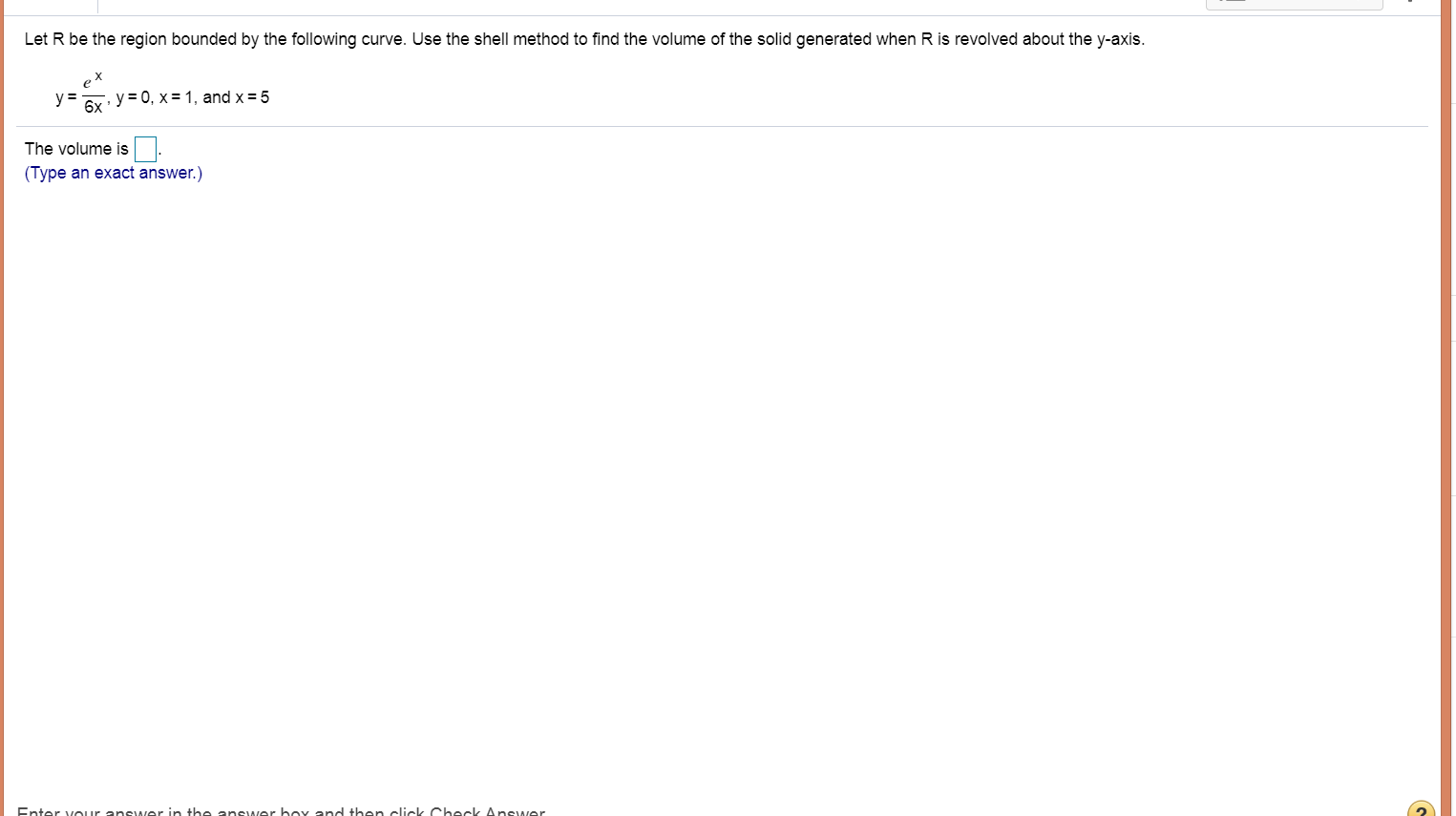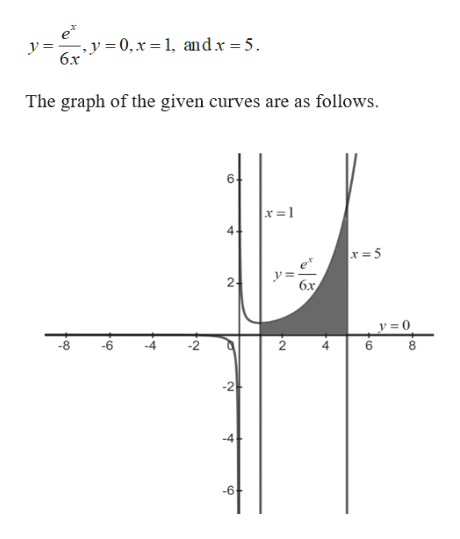# Let R be the region bounded by the following curve. Use the shell method to find the volume of the solid generated when R is revolved about the y-axisy=6x y 0, x 1, and x = 5The volume is(Type an exact answer.)Enter your answer in the answer boy and then click Check Answer

Question
1 viewshelp_outlineImage TranscriptioncloseLet R be the region bounded by the following curve. Use the shell method to find the volume of the solid generated when R is revolved about the y-axis y= 6x y 0, x 1, and x = 5 The volume is (Type an exact answer.) Enter your answer in the answer boy and then click Check Answer fullscreen
check_circle

star
star
star
star
star
1 Rating
Step 1

The given curve are,

Step 2

The given curv...help_outlineImage Transcriptionclosee y=. y = 0, x = 1, and x = 5. 6x The graph of the given curves are as follows. 6 x =1 4- x =5 y= бх y = 0 -8 -6 2 8 -4 -6 st 2 fullscreen

### Want to see the full answer?

See Solution

#### Want to see this answer and more?

Solutions are written by subject experts who are available 24/7. Questions are typically answered within 1 hour.*

See Solution
*Response times may vary by subject and question.
Tagged in

### Other